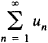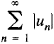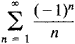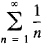# Conditional Convergence

(redirected from Conditionally convergent)
Also found in: Dictionary.
Related to Conditionally convergent: absolutely convergent, Absolutely convergent series

## conditional convergence

[kən′dish·ən·əl kən′vər·jəns]
(mathematics)
The property of a series that is convergent but not absolutely convergent.

## Conditional Convergence

a concept of mathematical analysis. The seriesis said to be conditionally convergent if it is convergent and the series(whose terms are the absolute values of the terms of the original series) is divergent.

For example, the seriesis conditionally convergent, since the absolute values of its terms form the divergent, harmonic seriesIf a series is conditionally convergent, then the two series consisting of its positive and negative terms, respectively, are divergent. According to Riemann’s theorem, by an appropriate rearrangement of the terms of a given conditionally convergent series we can obtain a divergent series or a series that has a prescribed sum. If two conditionally convergent series are multiplied term by term, a divergent series may result.

The concept of conditional convergence can be extended to series of vectors, to infinite products, and to improper integrals.

Site: Follow: Share:
Open / Close